x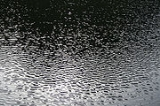Capillary waveEncyclopedia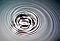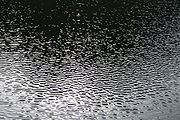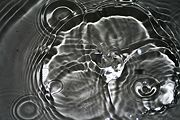A capillary wave is a wave
Wave
In physics, a wave is a disturbance that travels through space and time, accompanied by the transfer of energy.Waves travel and the wave motion transfers energy from one point to another, often with no permanent displacement of the particles of the medium—that is, with little or no associated mass...

traveling along the phase boundary
Phase boundary
The behavior of phase boundaries has been a developing subject of interest and an active research field in physics and mathematics for almost two centuries. One reason behind this is that phase boundaries naturally arise in many physical processes due to immiscibility of two or more substances with...

of a fluid, whose dynamics
Dynamics (mechanics)
In the field of physics, the study of the causes of motion and changes in motion is dynamics. In other words the study of forces and why objects are in motion. Dynamics includes the study of the effect of torques on motion...

are dominated by the effects of surface tension
Surface tension
Surface tension is a property of the surface of a liquid that allows it to resist an external force. It is revealed, for example, in floating of some objects on the surface of water, even though they are denser than water, and in the ability of some insects to run on the water surface...

.

Capillary waves are common in nature
Nature
Nature, in the broadest sense, is equivalent to the natural world, physical world, or material world. "Nature" refers to the phenomena of the physical world, and also to life in general...

and the home, and are often referred to as ripples. The wavelength
Wavelength
In physics, the wavelength of a sinusoidal wave is the spatial period of the wave—the distance over which the wave's shape repeats.It is usually determined by considering the distance between consecutive corresponding points of the same phase, such as crests, troughs, or zero crossings, and is a...

of capillary waves in water is typically less than a few cm.

When generated by light wind in open water, a nautical name for them is "cat's paw" waves, since they may resemble paw prints. Light breezes which stir up such small ripples are also sometimes referred to as cat's paws. On the open ocean, much larger ocean surface waves (seas and swell
Swell (ocean)
A swell, in the context of an ocean, sea or lake, is a series surface gravity waves that is not generated by the local wind. Swell waves often have a long wavelength but this varies with the size of the water body, e.g. rarely more than 150 m in the Mediterranean, and from event to event, with...

s) may result from coalescence of smaller wind-caused ripple-waves.

A gravity–capillary wave on a fluid interface is influenced by both the effects of surface tension and gravity
Standard gravity
Standard gravity, or standard acceleration due to free fall, usually denoted by g0 or gn, is the nominal acceleration of an object in a vacuum near the surface of the Earth. It is defined as precisely , or about...

, as well as by fluid inertia
Inertia
Inertia is the resistance of any physical object to a change in its state of motion or rest, or the tendency of an object to resist any change in its motion. It is proportional to an object's mass. The principle of inertia is one of the fundamental principles of classical physics which are used to...

.

## Capillary waves, proper

The dispersion relation
Dispersion relation
In physics and electrical engineering, dispersion most often refers to frequency-dependent effects in wave propagation. Note, however, that there are several other uses of the word "dispersion" in the physical sciences....

for capillary waves is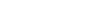where ω is the angular frequency
Angular frequency
In physics, angular frequency ω is a scalar measure of rotation rate. Angular frequency is the magnitude of the vector quantity angular velocity...

, σ the surface tension
Surface tension
Surface tension is a property of the surface of a liquid that allows it to resist an external force. It is revealed, for example, in floating of some objects on the surface of water, even though they are denser than water, and in the ability of some insects to run on the water surface...

, ρ the density
Density
The mass density or density of a material is defined as its mass per unit volume. The symbol most often used for density is ρ . In some cases , density is also defined as its weight per unit volume; although, this quantity is more properly called specific weight...

of the
heavier fluid, ρ the density of the lighter fluid and k the wavenumber
Wavenumber
In the physical sciences, the wavenumber is a property of a wave, its spatial frequency, that is proportional to the reciprocal of the wavelength. It is also the magnitude of the wave vector...

. The wavelength
Wavelength
In physics, the wavelength of a sinusoidal wave is the spatial period of the wave—the distance over which the wave's shape repeats.It is usually determined by considering the distance between consecutive corresponding points of the same phase, such as crests, troughs, or zero crossings, and is a...

is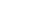## Gravity–capillary waves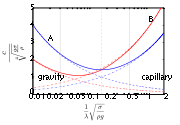In general, waves are also affected by gravity and are then called gravity–capillary waves. Their dispersion relation reads, for waves on the interface between two fluids of infinite depth: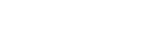where g is the acceleration due to gravity
Standard gravity
Standard gravity, or standard acceleration due to free fall, usually denoted by g0 or gn, is the nominal acceleration of an object in a vacuum near the surface of the Earth. It is defined as precisely , or about...

, ρ and ρ‘ are the mass density of the two fluids (ρ > ρ‘). Notice the factor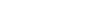in the first term is the Atwood number.

### Gravity wave regime

For large wavelengths (small k = 2π/λ), only the first term is relevant and one has gravity wave
Gravity wave
In fluid dynamics, gravity waves are waves generated in a fluid medium or at the interface between two media which has the restoring force of gravity or buoyancy....

s.
In this limit, the waves have a group velocity
Group velocity
The group velocity of a wave is the velocity with which the overall shape of the wave's amplitudes — known as the modulation or envelope of the wave — propagates through space....

half the phase velocity
Phase velocity
The phase velocity of a wave is the rate at which the phase of the wave propagates in space. This is the speed at which the phase of any one frequency component of the wave travels. For such a component, any given phase of the wave will appear to travel at the phase velocity...

: following a single wave's crest in a group one can see the wave appearing at the back of the group, growing and finally disappearing at the front of the group.

### Capillary wave regime

Shorter (large k) waves (e.g. 2 mm), which are proper capillary waves, do the opposite: an individual wave appears at the front of the group, grows when moving towards the group center and finally disappears at the back of the group. Phase velocity is two thirds of group velocity in this limit.

### Phase velocity minimum

Between these two limits, an interesting and common situation occurs when the dispersion caused by gravity cancels out the dispersion due to the capillary effect. At a certain wavelength, the group velocity equals the phase velocity, and there is no dispersion. At precisely this same wavelength, the phase velocity of gravity–capillary waves as a function of wavelength (or wave number) has a minimum. Waves with wavelengths much smaller than this critical wavelength λc are dominated by surface tension, and much above by gravity. The value of this wavelength is: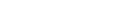For the air–water
Water
Water is a chemical substance with the chemical formula H2O. A water molecule contains one oxygen and two hydrogen atoms connected by covalent bonds. Water is a liquid at ambient conditions, but it often co-exists on Earth with its solid state, ice, and gaseous state . Water also exists in a...

interface, λc is found to be 1.7 cm.

### Derivation

As Richard Feynman
Richard Feynman
Richard Phillips Feynman was an American physicist known for his work in the path integral formulation of quantum mechanics, the theory of quantum electrodynamics and the physics of the superfluidity of supercooled liquid helium, as well as in particle physics...

put it, "[water waves] that are easily seen by everyone and which are usually used as an example of waves in elementary courses [...] are the worst possible example [...]; they have all the complications that waves can have." The derivation of the general dispersion relation is therefore quite involved.

Therefore, first the assumptions involved are pointed out. There are three contributions to the energy, due to gravity, to surface tension
Surface tension
Surface tension is a property of the surface of a liquid that allows it to resist an external force. It is revealed, for example, in floating of some objects on the surface of water, even though they are denser than water, and in the ability of some insects to run on the water surface...

, and to hydrodynamics. The first two are potential energies, and responsible for the two terms inside the parenthesis, as is clear from the appearance of g and σ. For gravity, an assumption is made of the density of the fluids being constant (i.e., incompressibility), and likewise g (waves are not high for gravitation to change appreciably). For surface tension, the deviations from planarity (as measured by derivatives of the surface) are supposed to be small. Both approximations are excellent for common waves.

The last contribution involves the kinetic energies
Kinetic energy
The kinetic energy of an object is the energy which it possesses due to its motion.It is defined as the work needed to accelerate a body of a given mass from rest to its stated velocity. Having gained this energy during its acceleration, the body maintains this kinetic energy unless its speed changes...

of the fluids, and is the most involved. One must use a hydrodynamic framework to tackle this problem. Incompressibility is again involved (which is satisfied if the speed of the waves is much less than the speed of sound in the media), together with the flow being irrotational – the flow is then
potential
Potential flow
In fluid dynamics, potential flow describes the velocity field as the gradient of a scalar function: the velocity potential. As a result, a potential flow is characterized by an irrotational velocity field, which is a valid approximation for several applications...

; again, these are typically good approximations for common situations. The resulting equation for the potential (which is Laplace equation) can be solved with the proper boundary conditions. On one hand, the velocity must vanish well below the surface (in the "deep water" case, which is the one we consider, otherwise a more involved result is obtained, see Ocean surface waves.) On the other, its vertical component must match the motion of the surface. This contribution ends up being responsible for the extra k outside the parenthesis, which causes all regimes to be dispersive, both at low values of k, and high ones (except around the one value at which the two dispersions cancel out.)

• Capillary action
Capillary action
Capillary action, or capilarity, is the ability of a liquid to flow against gravity where liquid spontanously rise in a narrow space such as between the hair of a paint-brush, in a thin tube, or in porous material such as paper or in some non-porous material such as liquified carbon fiber, or in a...

• Dispersion (water waves)
Dispersion (water waves)
In fluid dynamics, dispersion of water waves generally refers to frequency dispersion, which means that waves of different wavelengths travel at different phase speeds. Water waves, in this context, are waves propagating on the water surface, and forced by gravity and surface tension...

• Fluid pipe
• Thermal capillary wave
Thermal capillary wave
Thermal motion is able to produce capillary waves at the molecular scale. At this scale,gravity and hydrodynamics can be neglected, and only the surface tension contribution isrelevant....

• Two-phase flow
• Ocean surface wave
Ocean surface wave
In fluid dynamics, wind waves or, more precisely, wind-generated waves are surface waves that occur on the free surface of oceans, seas, lakes, rivers, and canals or even on small puddles and ponds. They usually result from the wind blowing over a vast enough stretch of fluid surface. Waves in the...

• Wave-formed ripple